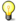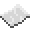# math.sinNote: The argument must be given in radians. If it is in degrees, use the math.rad function to convert it.ExamplePrint the sine of user input
Code
```<nowiki>
</nowiki>
```
Output Depends on what the user wrote. For instance, if they were to enter `90`, the number printed would be `1`.

math.sin
Function
Syntax
math.sin(
• num : number
)

Returns number
API math
Source Lua (source)

Returns the sine of num.